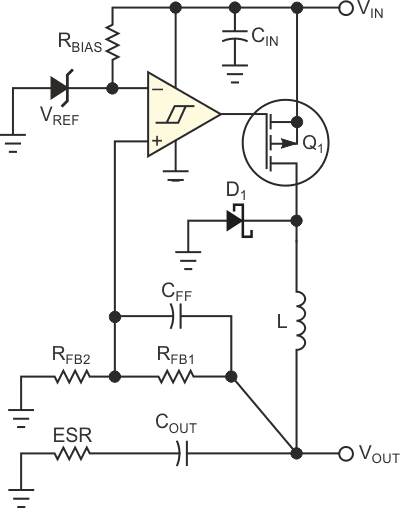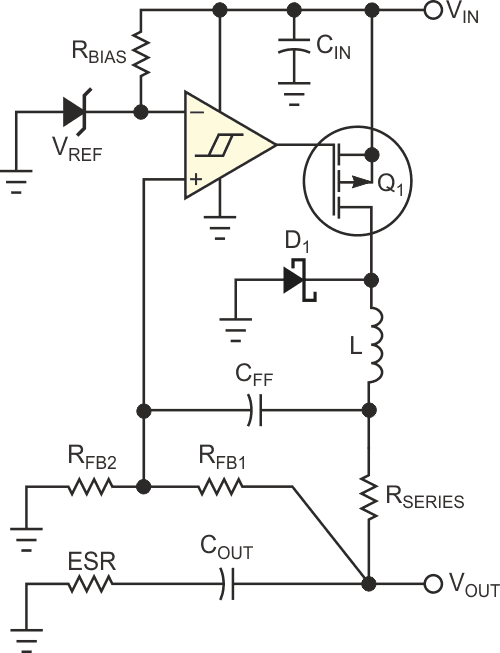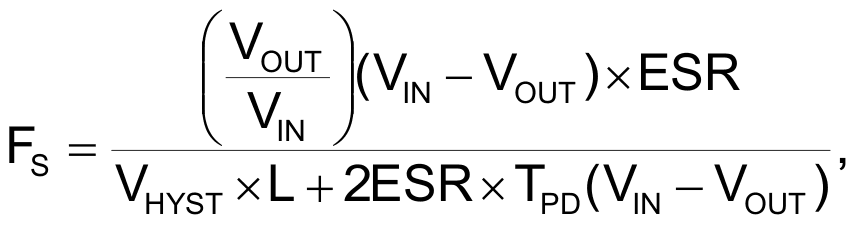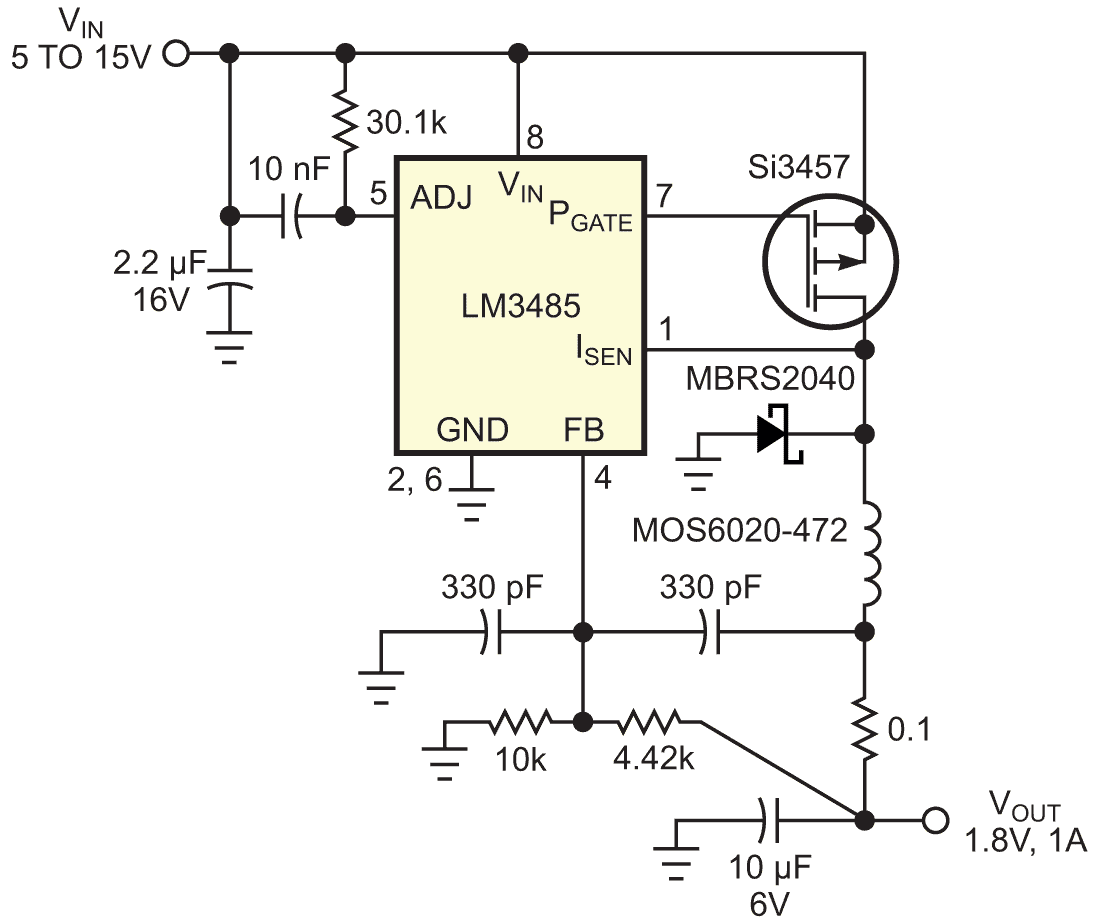# Hysteretic regulators provide high performance at low cost

## Texas Instruments LM3485 LMV7219

Hysteretic voltage regulators offer the potential advantages of simplicity, fast response, 100%-duty-cycle operation, high efficiency at light loading, and low cost. They need no loop-compensation components to add delays; thus, response time to a load change is less than one switching cycle. What's the catch? You must be able to accept a switching frequency that is not precisely controlled and a sensitivity to noise that requires layout skill.

Figure 1 shows a simple hysteretic switching regulator made from a comparator with a fixed hysteresis and a PFET. The comparator switches on the PFET whenever VOUT falls to its low threshold and off again when VOUT rises to its high threshold. The time VOUT lingers between the thresholds determines the on-time and, hence, the switching frequency. The inductor's ripple current flowing through the ESR of COUT provides a triangular voltage-ripple waveform, which produces unpredictable operation.Figure 1. This hysteretic regulator suffers from unpredictability of the switching frequency because of COUT ESR variance.

Herein lies a potential problem with simple circuits of this type. ESR is a major factor in determining switching frequency, and ESR can vary over a wide range for any given capacitor type. This variance is seldom a good thing and can lead to inductor saturation if the frequency falls too low or FET overheating arising from switching losses if the frequency rises too high. A simple solution to the ESR-variance problem is to use a ceramic COUT capacitor in series with a resistor. Although this technique works nicely in the lab, it often poses problems in the real world, in which several ceramic capacitors bypass loads.

Another approach to predictable frequency control allows the use of low-ESR capacitors (Figure 2). It is almost identical to Figure 1's circuit except for the added resistor, RSERIES, and the new connection point for CFF. The inductor's ripple current induces the ac voltage present across RSERIES and connects to comparator by CFF. This controlled ac voltage eliminates the need for any COUT ESR. The feedback loop eliminates the dc voltage drop that RSERIES creates. This new configuration produces predictable switching frequency with even zero-ESR capacitors and offers the potential of nearly zero VOUT ripple at the cost of a resistor and the small added dissipation of RSERIES carrying full load current.Figure 2. An added series resistor makes this circuit’s switching frequency more predictable.

The following equation approximates the switching frequency for either circuit, provided that COUT’s reactance at the switching frequency is lower than the ESR and CFF’s reactance is much lower than RFB1:where ESR is the sum of COUT’s ESR and RSERIES, VHYST is the comparator's hysteresis voltage, and TPD is the average propagation delay of the comparator plus the PFET.

You can build the circuits of Figure 1 and Figure 2 as drawn, using a comparator, such as the LMV7219, which claims 7.5-mV built-in hysteresis, or by using a controller, such as the LM3485, which provides a current-limiting feature, wider VIN range, and lower cost. You cannot overemphasize the layout sensitivity for hysteretic regulators. You cannot allow the feedback connection to pick up any stray signals. Open-core inductors are attractive for cost reasons but difficult to use, because any induced voltages from stray magnetic fields can produce unpredictable switching frequencies and ripple.Figure 3. This circuit occupies an area smaller than a postage stamp.

You can build the circuit in Figure 3 in an area smaller than a postage stamp. This circuit produces output current of at least 1 A, using small ceramic capacitors, a TSOP-6 PFET, a 6×7-mm inductor, and an SMB-package, surface-mount Schottky diode. FS varies from 600 to 700 kHz over a VIN range of 5 to 15 V for VOUT = 1.8 V and VOUT ripple less than 5 mV p-p. The 30.1-kΩ resistor and the PFET's on-resistance of 0.1 Ω set the current limit to trigger at 1.5 A. The no-load bias current is lower than 500 µA. Most impressive is the dynamic VOUT change of only 10 mV for a load transient greater than 0.5 A.

EDN